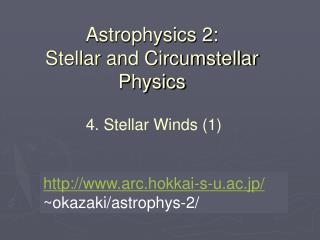DownloadDownload PresentationAstrophysics 2: Stellar and Circumstellar Physics

# Astrophysics 2: Stellar and Circumstellar Physics

Télécharger la présentation## Astrophysics 2: Stellar and Circumstellar Physics

- - - - - - - - - - - - - - - - - - - - - - - - - - - E N D - - - - - - - - - - - - - - - - - - - - - - - - - - -
##### Presentation Transcript

1. Astrophysics 2:Stellar and Circumstellar Physics 4. Stellar Winds (1) http://www.arc.hokkai-s-u.ac.jp/ ~okazaki/astrophys-2/

2. 4.1.1 Solar wind 4.1 Observations of stellar winds • Radiative core • Convective envelope, where dynamo process is going on • Corona, where the solar wind begins to blow Structure of the Sun

3. Northern lights

4. Boundary between the solar system and the interstellar space

5. Why the solar wind blows? (Parker 1958) Suppose the solar corona is static, then the equation of motion is given by If we assume the corona to be isothermal, i.e., with being the isothermal sound speed, we have

6. where Therefore, the solar corona can’t be static.

7. 4.1.2 Winds from massive stars: P Cygni profiles P Cygni profile: Profile characterized by strong emission lines with corresponding blueshifted absorption lines.

8. P Cygni profiles: lines from an expanding atmosphere/stellar wind Emission Absorption E E A Total observer wavelength

9. http://casswww.ucsd.edu/public/tutorial/Stars.html

10. Formation of a P Cygni Line- Profile By S. Owocki

11. 4.2 General equations and formalism for stellar winds 4.2.1 What is a stellar wind? • A stellar wind is: • a sustained outflow in the outer layers of a star, through which the star loses its mass continuously. • a source of mass, angular momentum, and energy to the interstellar matter.

12. 4.2.2 Hydrostatic equilibrium in the base of a wind Eq of motion: Eq of state: T varies gradually

13. In the base of a wind, the atmosphere is exponentially stratified with a scale height much smaller than the stellar radius. e.g., Solar photosphere

14. 4.2.3. General dynamical equations Mass Momentum Internal energy EOS

15. Steady, spherical expansion Mass loss rate Momentum Total energy work heating conduction

16. kinetic energy potential energy Energy requirement work heating conduction

17. Driving mechanism of coronal winds = gas pressure gradient 4.2.4 A simple model of coronal wind: an isothermal wind Assumptions • Steady & spherically symmetric. • Forces taken into account are only gravity and pressure gradient force.

18. Coronal wind Corona heating Convective envelope Coronal winds are driven by gas pressure due to a high T in the corona.

19. Wind eq: Basic equations Eq of continuity: Eq of motion: Eq of state:

20. Wind eq has a singularity at • The critical point is at • The critical point is of saddle type (x-type), which is stable for perturbations • At the critical point, (sonic point),

21. Solution curves for an isothermal coronal wind (transonic solution)

22. 4.2.5 Temperature sensitivity of mass loss rate At the bottom of a subsonic wind with we have

23. The density distribution is

24. Mass loss rate is very sensitive to the temperature! Mass loss rate

25. Mass loss rate vs. temperature (Owocki 2000)

26. 4.3 Analogy of De laval nozzles Critical solutions have an analogy with flows in rocket nozzles.

27. Basic equations Eq of continuity: Eq of motion: Eq of state: Flow eq:

28. Wind eq: Flow eq: Both equations would be identical if